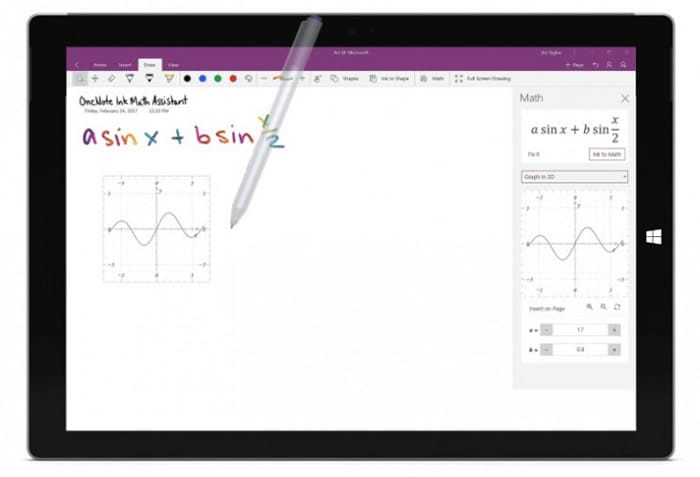# OneNote for Windows 10 already allows graphing mathematical equationsThe new OneNote update for Windows 10 has brought a series of new features, but we highlight a very useful feature for those who want extra help for their math assignments.

In this version OneNote extends to all the function that allows graphing mathematical equations with just a few clicks and selecting some options. The procedure is very simple, we just have to write the equation, go to the Draw section and with the selection of loop choose it and click on the Math icon >> 2D graphic.

Following this dynamic will appear an interactive graphic, that we can change its position with a few simple touches or simply drag and drop. We can also change the values of the equation, and see how the graph settings are automatically modified.

We can see an example of the operation of this new function in this image published by Microsoft which you can see above.

In the blog of Microsoft they share the step by step how to graph equations using OneNote, so we can take a look at this link.

And if you do not have OneNote installed, or prefer to try other options and resources to graph, you can take into account different alternatives that allow us to solve and graph mathematical problems.
This feature provided by Microsoft in the OneNote tool will help many students to get help with their mathematical equations. Try this tool and let us know about your experience.